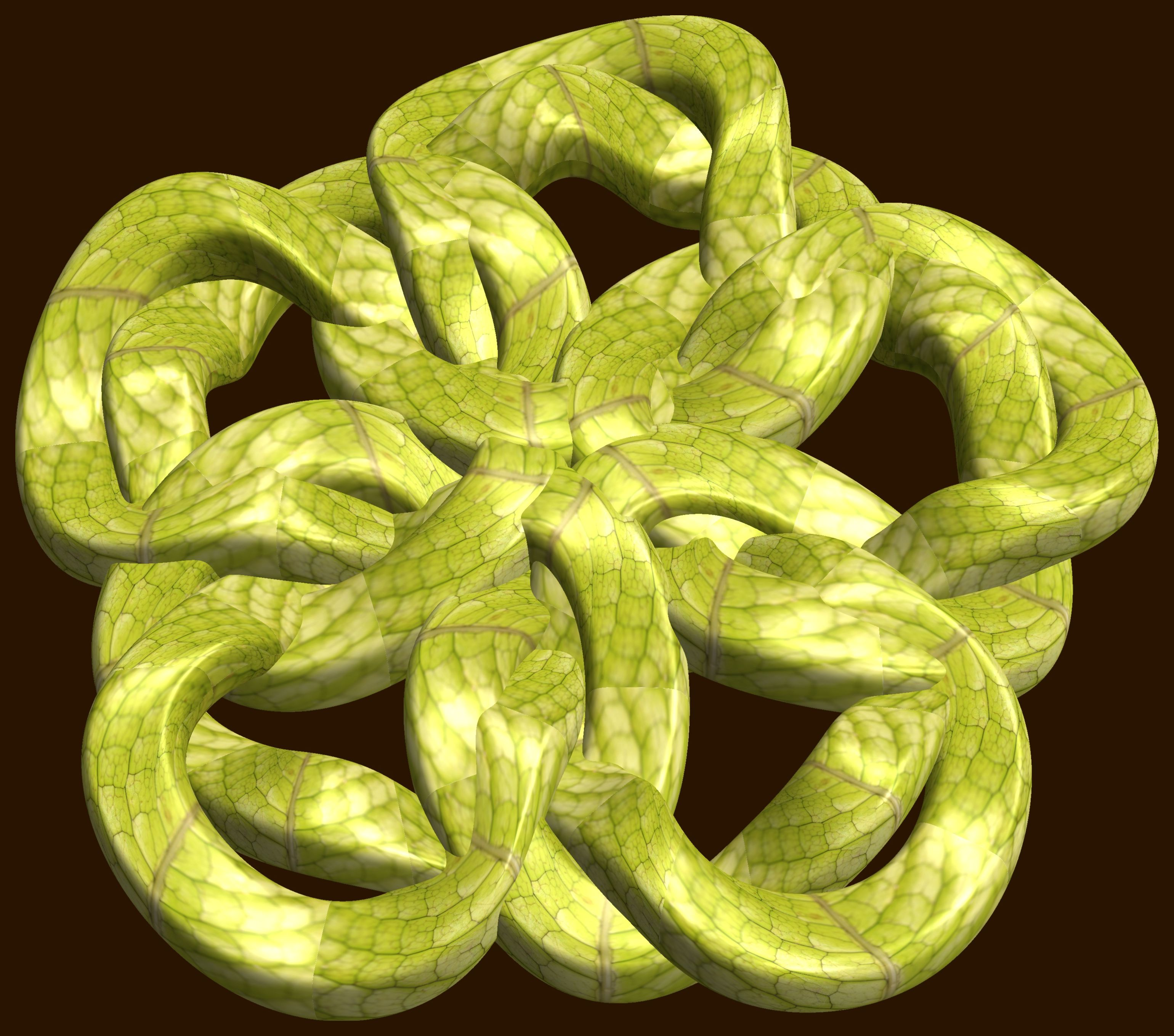# 5 Knot-Tori / ５個の結び目

###### Related for 5 Knot-Tori / ５個の結び目5 knot-tori / ５個の結び目 free stock photo

(* === Following code of Mathematica 8 generates this image. === *)

a = 4; (* center hole size of a torus *)

b1 = 3; (* number of cross *)

b2 = 2; (* number of cross *)

b3 = 5; (* number of cross *)

c = 4; (* distance from the center of rotation *)

d = 5; (* number of torus *)

h = 1; (* height of a torus *)

SetOptions[ParametricPlot3D, PlotRange -> Full, Mesh -> None,

Boxed -> False, Axes -> None, PlotPoints -> 500, ImageSize -> 3000,

Background -> RGBColor[{40, 20, 0}/255],

PlotStyle -> Directive[Specularity[White, 90], Texture[Import["D:/tmp/71.jpg"]]],

TextureCoordinateFunction -> ({#4, #5 b1 Pi} &), Lighting -> "Neutral"];

f[v_] := Sin[2 Sin[Sin[Sin[v]]]];

x = (a - Cos[t] + f[b1 s]) Cos[b2 s] + c;

y = (a - Cos[t] + f[b1 s]) Sin[b2 s] + c;

z = h (Sin[b3 (s + Pi/2)] + f[t]) + c;

rm = Table[{x, y, z}.RotationMatrix[2 i Pi/d, {0, 0, 1}], {i, d}];

ParametricPlot3D[rm, {t, 0, 2 Pi}, {s, 0, 2 Pi}]

(*---

Another shapes and colors

*)

Free high resolution images 5 knot-tori / ５個の結び目, 3d, デザイン, むすびめ, もよう, アブストラクト.
Licence: Creative Commons - CC0. Photos transferred to the public domain from Creative Commons.

###### Tags: